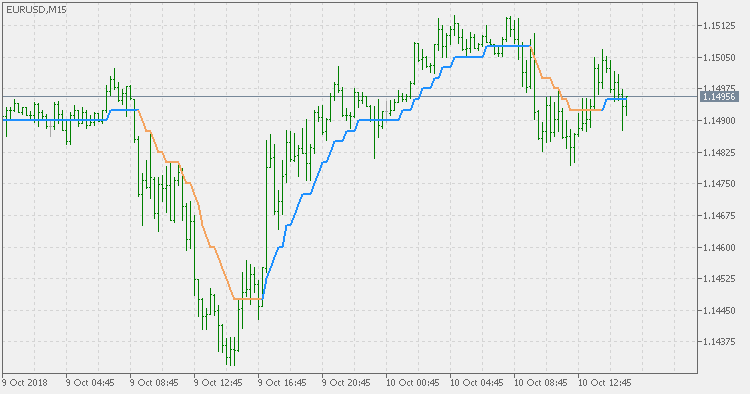Interesting script?
So post a link to it -
let others appraise it

You liked the script? Try it in the MetaTrader 5 terminal# Step average - indicator for MetaTrader 5

Views:
2071
Rating:
Published:
2018.10.10 15:01
Updated:
2019.01.29 14:14

Basics:

We are all using averages for market assessment. One of the ways averages are used is using the change of the slope of the average as a signal. But that way tends to produce a lot of signals in ranging market. One of the way to lessen that number of signals is to filter out insignificant changes in average.

This version:

It is using fixed "step" change in pips as a filter. If the average does not changes for more than the required step, the value of the average remains the same. Otherwise it is changed in order to reflect the nearest value based on the required steps. Averages supported are the following:

• simple moving average (SMA)
• exponential moving average (EMA)
• smoothed moving average (SMMA)
• linear weighted moving average (LWMA)

Usage:

You can use the color changes as signals.Stochastic volatility - on chart

Stochastic volatility - on chartVidya

Variable Index Dynamic Average (VIDYA)Step average (atr based)

Step average - average true range basedStep average - std based

Step average - standard deviation based